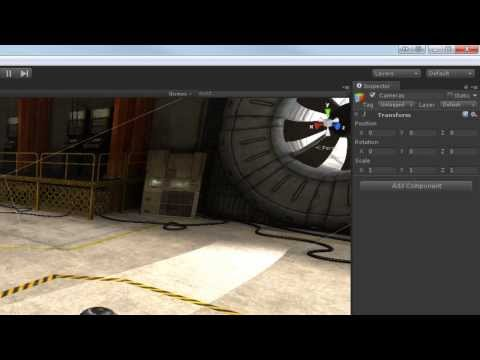# Quaternions

#### Dificuldade: Intermediário

How to utilize the quaternion system to manage the rotation of game objects.## Quaternions

Intermediário Scripting

### Code snippet

``````using UnityEngine;
using System.Collections;

public class MotionScript : MonoBehaviour
{
public float speed = 3f;

void Update ()
{
transform.Translate(-Input.GetAxis("Horizontal") * speed * Time.deltaTime, 0, 0);
}
}``````
``````#pragma strict

public var speed : float = 3f;

function Update ()
{
transform.Translate(-Input.GetAxis("Horizontal") * speed * Time.deltaTime, 0, 0);
}``````
``````import UnityEngine
import System.Collections

public class MotionScript(MonoBehaviour):

public speed as single = 3.0F

private def Update():
transform.Translate((((-Input.GetAxis('Horizontal')) * speed) * Time.deltaTime), 0, 0)``````

### Code snippet

``````using UnityEngine;
using System.Collections;

public class LookAtScript : MonoBehaviour
{
public Transform target;

void Update ()
{
Vector3 relativePos = target.position - transform.position;
transform.rotation = Quaternion.LookRotation(relativePos);
}
}``````
``````#pragma strict

public var target : Transform;

function Update ()
{
var relativePos : Vector3 = target.position - transform.position;
transform.rotation = Quaternion.LookRotation(relativePos);
}``````
``````import UnityEngine
import System.Collections

public class LookAtScript(MonoBehaviour):

public target as Transform

private def Update():
relativePos as Vector3 = (target.position - transform.position)
transform.rotation = Quaternion.LookRotation(relativePos)``````

### Code snippet

``````using UnityEngine;
using System.Collections;

public class GravityScript : MonoBehaviour
{
public Transform target;

void Update ()
{
Vector3 relativePos = (target.position + new Vector3(0, 1.5f, 0)) - transform.position;
Quaternion rotation = Quaternion.LookRotation(relativePos);

Quaternion current = transform.localRotation;

transform.localRotation = Quaternion.Slerp(current, rotation, Time.deltaTime);
transform.Translate(0, 0, 3 * Time.deltaTime);
}
}``````
``````#pragma strict

public var target : Transform;

function Update ()
{
var relativePos : Vector3 = (target.position + new Vector3(0, 1.5f, 0)) - transform.position;
var rotation : Quaternion = Quaternion.LookRotation(relativePos);

var current : Quaternion = transform.localRotation;

transform.localRotation = Quaternion.Slerp(current, rotation, Time.deltaTime);
transform.Translate(0, 0, 3 * Time.deltaTime);
}``````
``````import UnityEngine
import System.Collections

public class GravityScript(MonoBehaviour):

public target as Transform

private def Update():
relativePos as Vector3 = ((target.position + Vector3(0, 1.5F, 0)) - transform.position)
rotation as Quaternion = Quaternion.LookRotation(relativePos)

current as Quaternion = transform.localRotation

transform.localRotation = Quaternion.Slerp(current, rotation, Time.deltaTime)
transform.Translate(0, 0, (3 * Time.deltaTime))``````

### Code snippet

``````using UnityEngine;
using System.Collections;

public class SomeClass : MonoBehaviour
{
void Start ()
{
transform.rotation = Quaternion.identity;
}
}``````
``````#pragma strict

function Start ()
{
transform.rotation = Quaternion.identity;
}``````
``````import UnityEngine
import System.Collections

public class SomeClass(MonoBehaviour):

private def Start():
transform.rotation = Quaternion.identity``````